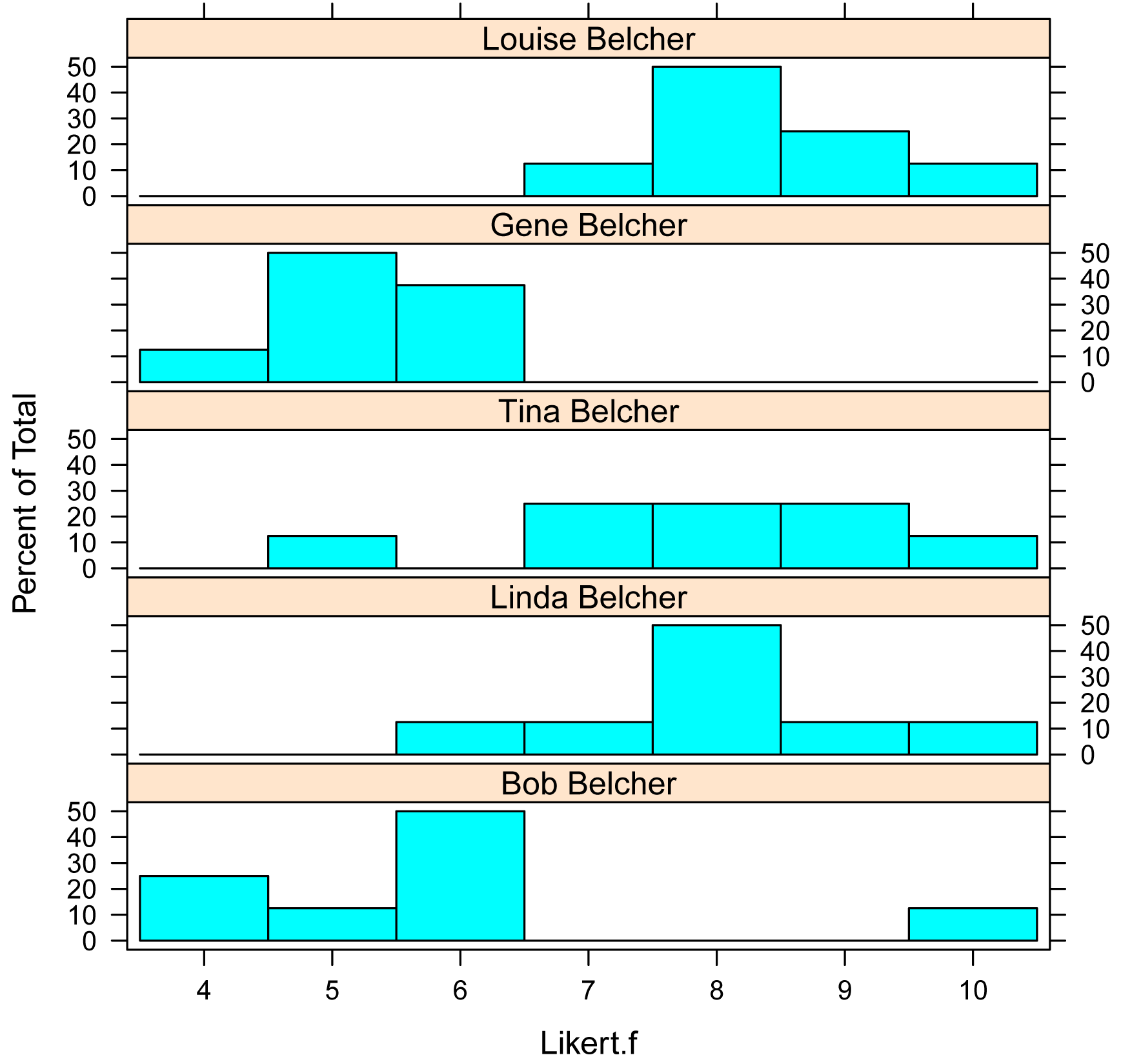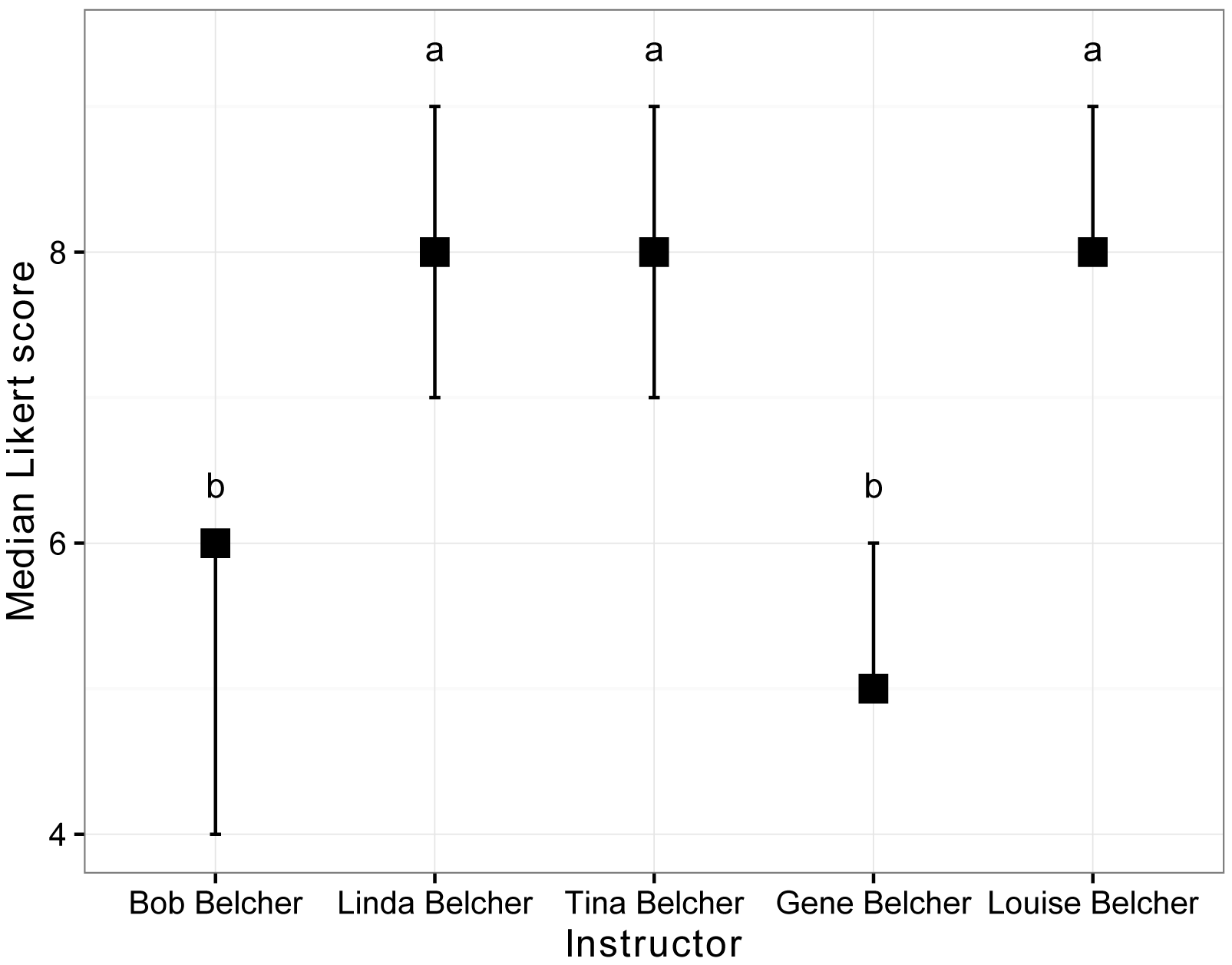## Summary and Analysis of Extension Program Evaluation in R

Salvatore S. Mangiafico

# One-way Permutation Test of Symmetry for Paired Ordinal Data

### When to use this test

A permutation test of symmetry can be used for one-way data with an ordinal dependent variable where observations are paired within a blocking variable.  It will determine if there is a difference in the response variable among groups when controlling for the effect of the blocking variable.  There can be two or more groups.

The coin package can accomodate designs used with Friedman, Quade, paired t-test, repeated measures one-way anova, and ordinal regression equivalents.

The test does not make assumptions about the distribution of values.

The test is performed with the symmetry_test function in the coin package.

Post-hoc testing can be conducted with pairwise permutation tests across groups.

It is important that the dependent variable be specified as an ordered factor variable in R, if it is to be treated as an ordinal variable.

##### Appropriate data

•  One-way data plus a blocking variable.  That is, one measurement variable in two or more groups, where observations are paired within levels of a blocking variable

•  Dependent variable is ordinal, and specified in R as an ordered factor variable

•  Independent variable is a factor with two or more levels.  That is, two or more groups.  The blocking variable is also a factor variable

•  The data is arranged in a complete block design, with one or more observations per cell

##### Hypotheses

•  Null hypothesis:  The response of the dependent variable among groups are equal.

•  Alternative hypothesis (two-sided): The response of the dependent variable among groups are not equal.

##### Interpretation

•  Reporting significant results for the omnibus test as “Significant differences were found in the response among groups.” is acceptable.  Alternatively, “A significant effect for Independent Variable on Dependent Variable was found when controlling for the effect of Blocking Variable.”

•  Reporting significant results for mean separation post-hoc tests as “Response of Dependent Variable for group A was different than that for group B.” is acceptable.

##### Other notes and alternative tests

Ordinal regression is an alternative.

The traditional nonparametric tests Friedman, Quade, Paired rank-sum test, or Sign test may be alternatives depending on the design of the experiment.

### Packages used in this chapter

The packages used in this chapter include:

•  psych

•  lattice

•  FSA

•  coin

•  rcompanion

•  multcompView

•  ggplot2

The following commands will install these packages if they are not already installed:

if(!require(psych)){install.packages("psych")}
if(!require(FSA)){install.packages("FSA")}
if(!require(lattice)){install.packages("lattice")}
if(!require(coin)){install.packages("coin")}
if(!require(rcompanion)){install.packages("rcompanion")}
if(!require(multcompView)){install.packages("multcompView")}
if(!require(ggplot2)){install.packages("ggplot2")}

### One-way ordinal permutation test of symmetry example

This example re-visits the Belcher data from the Friedman Test chapter.  Note that each instructor is rated by each of eight raters.  Because of this, we want to stratify the responses by Rater.

It answers the question, “Are the scores significantly different among the five speakers when controlling for the effect of the different raters?”

Note that a new variable, Likert.f, is created for the Likert scores as an ordered factor variable.  It is necessary that the dependent variable passed to symmetry_test be an ordered factor variable for it to be treated as an ordinal variable.

Input =("
Instructor        Rater  Likert
'Bob Belcher'        a      4
'Bob Belcher'        b      5
'Bob Belcher'        c      4
'Bob Belcher'        d      6
'Bob Belcher'        e      6
'Bob Belcher'        f      6
'Bob Belcher'        g     10
'Bob Belcher'        h      6
'Linda Belcher'      a      8
'Linda Belcher'      b      6
'Linda Belcher'      c      8
'Linda Belcher'      d      8
'Linda Belcher'      e      8
'Linda Belcher'      f      7
'Linda Belcher'      g     10
'Linda Belcher'      h      9
'Tina Belcher'       a      7
'Tina Belcher'       b      5
'Tina Belcher'       c      7
'Tina Belcher'       d      8
'Tina Belcher'       e      8
'Tina Belcher'       f      9
'Tina Belcher'       g     10
'Tina Belcher'       h      9
'Gene Belcher'       a      6
'Gene Belcher'       b      4
'Gene Belcher'       c      5
'Gene Belcher'       d      5
'Gene Belcher'       e      6
'Gene Belcher'       f      6
'Gene Belcher'       g      5
'Gene Belcher'       h      5
'Louise Belcher'     a      8
'Louise Belcher'     b      7
'Louise Belcher'     c      8
'Louise Belcher'     d      8
'Louise Belcher'     e      9
'Louise Belcher'     f      9
'Louise Belcher'     g      8
'Louise Belcher'     h     10
")

### Order levels of the factor; otherwise R will alphabetize them

Data\$Instructor = factor(Data\$Instructor,
levels=unique(Data\$Instructor))

### Create a new variable which is the likert scores as an ordered factor

Data\$Likert.f = factor(Data\$Likert,
ordered=TRUE)

###  Check the data frame

library(psych)

str(Data)

summary(Data)

### Remove unnecessary objects

rm(Input)

#### Summarize data treating Likert scores as factors

Note that the variable we want to count is Likert.f, which is a factor variable.  Counts for Likert.f are cross tabulated over values of Instructor.  The prop.table function translates a table into proportions.  The margin=1 option indicates that the proportions are calculated for each row.

xtabs( ~ Instructor + Likert.f,
data = Data)

Likert.f
Instructor       4 5 6 7 8 9 10
Bob Belcher    2 1 4 0 0 0  1
Linda Belcher  0 0 1 1 4 1  1
Tina Belcher   0 1 0 2 2 2  1
Gene Belcher   1 4 3 0 0 0  0
Louise Belcher 0 0 0 1 4 2  1

XT = xtabs( ~ Instructor + Likert.f,
data = Data)

prop.table(XT,
margin = 1)

Likert.f
Instructor           4     5     6     7     8     9    10
Bob Belcher    0.250 0.125 0.500 0.000 0.000 0.000 0.125
Linda Belcher  0.000 0.000 0.125 0.125 0.500 0.125 0.125
Tina Belcher   0.000 0.125 0.000 0.250 0.250 0.250 0.125
Gene Belcher   0.125 0.500 0.375 0.000 0.000 0.000 0.000
Louise Belcher 0.000 0.000 0.000 0.125 0.500 0.250 0.125

#### Bar plots by group

Note that the variable we want to count is Likert.f, which is a factor variable.  Counts for Likert.f are presented for values of Speaker.  Also note that the histograms don’t show the effect of the blocking variable.

library(lattice)

histogram(~ Likert.f | Instructor,
data=Data,
layout=c(1,5))      #  columns and rows of individual plots#### Summarize data treating Likert scores as numeric

It may be useful to look at the minimum, first quartile, median, third quartile, and maximum for Likert for each group.

library(FSA)

Summarize(Likert ~ Instructor,
data=Data,
digits=3)

Instructor n  mean    sd min   Q1 median   Q3 max percZero
1    Bob Belcher 8 5.875 1.885   4 4.75      6 6.00  10        0
2  Linda Belcher 8 8.000 1.195   6 7.75      8 8.25  10        0
3   Tina Belcher 8 7.875 1.553   5 7.00      8 9.00  10        0
4   Gene Belcher 8 5.250 0.707   4 5.00      5 6.00   6        0
5 Louise Belcher 8 8.375 0.916   7 8.00      8 9.00  10        0

#### Permutation symmetry test

Note that the dependent variable is an ordered factor variable, Likert.fInstructor is the independent variable, and Rater is the blocking variable.  The data= option indicates the data frame that contains the variables.  For the meaning of other options, see library(coin); ?symmetry_test.

library(coin)

symmetry_test(Likert.f ~ Instructor | Rater,
data = Data)

Asymptotic General Symmetry Test

maxT = 3.4036, p-value = 0.003416

#### Post-hoc test: pairwise permutation tests

If the symmetry test is significant, a post-hoc analysis can be performed to determine which groups differ from which other groups.

The pairwisePermutationSymmetry and pairwisePermutationSymmetryMatrix functions in the rcompanion package conduct permutation tests across groups in a pairwise manner.   See library(rcompanion); ?pairwisePermutationSymmetry for further details.

Because the post-hoc test will produce multiple p-values, adjustments to the p-values can be made to avoid inflating the possibility of making a type-I error.  Here, the method of adjustment is indicated with the method option.  There are a variety of methods for controlling the familywise error rate or for controlling the false discovery rate.  See ?p.adjust for details on these methods.

Before conducting the pairwise tests, we will re-order the levels of the grouping variable by the median of each group.  This makes interpretation of the pairwise comparisons and compact letter display easier.

##### Table output and compact letter display

### Order groups by median

Data\$Instructor = factor(Data\$Instructor,
levels = c("Linda Belcher", "Louise Belcher",
"Tina Belcher", "Bob Belcher",
"Gene Belcher"))

### Pairwise permutation tests

library(rcompanion)

PT = pairwisePermutationSymmetry(Likert.f ~ Instructor | Rater,
data   = Data,
method = "fdr")

PT

1  Linda Belcher - Louise Belcher = 0 -0.9045   0.3657  0.42680
2    Linda Belcher - Tina Belcher = 0   0.378   0.7055  0.70550
3     Linda Belcher - Bob Belcher = 0   -2.38  0.01729  0.03458
4    Linda Belcher - Gene Belcher = 0   2.593 0.009522  0.03458
5   Louise Belcher - Tina Belcher = 0  -1.155   0.2482  0.35460
6    Louise Belcher - Bob Belcher = 0  -2.265  0.02354  0.03923
7   Louise Belcher - Gene Belcher = 0  -2.744 0.006068  0.03458
8      Tina Belcher - Bob Belcher = 0  -2.412  0.01586  0.03458
9     Tina Belcher - Gene Belcher = 0   2.528  0.01147  0.03458
10     Bob Belcher - Gene Belcher = 0  0.8704   0.3841  0.42680

### Compact letter display

library(rcompanion)

data = PT,
threshold  = 0.05)

Group Letter MonoLetter
1  LindaBelcher      a         a
2 LouiseBelcher      a         a
3   TinaBelcher      a         a
4    BobBelcher      b          b
5   GeneBelcher      b          b

Groups sharing a letter are not significantly different (alpha = 0.05).

##### Matrix output and compact letter display

A compact letter display condenses a table of p-values into a simpler format.  In the output, groups sharing a same letter are not significantly different.  Compact letter displays are a clear and succinct way to present results of multiple comparisons.

Here the fdr p-value adjustment method is used.  See ?p.adjust for details on available methods.

The code creates a matrix of p-values called PM which is then passed to the multcompLetters function to be converted to a compact letter display.

### Order groups by median

Data\$Instructor = factor(Data\$Instructor,
levels = c("Linda Belcher", "Louise Belcher",
"Tina Belcher", "Bob Belcher",
"Gene Belcher"))

### Pairwise permutation tests

library(rcompanion)

PM = pairwisePermutationSymmetryMatrix(Likert.f ~ Instructor | Rater,
data   = Data,
method = "fdr")

PM

# Produce compact letter display

library(multcompView)

compare="<",
threshold=0.05,  # p-value to use as significance threshold
Letters=letters,
reversed = FALSE)

Linda Belcher Louise Belcher   Tina Belcher    Bob Belcher   Gene Belcher
"a"            "a"            "a"            "b"            "b"

### Groups sharing a letter are not significantly different (alpha = 0.05).

#### Plot of medians and confidence intervals

The following code uses the groupwiseMedian function to produce a data frame of medians for each speaker along with the 95% confidence intervals for each median with the percentile method.  See library(rcompanion); ?groupwiseMedian for further options.

These medians are then plotted, with their confidence intervals shown as error bars.  The grouping letters from the multiple comparisons are added.

### Order groups by original order

Data\$Instructor = factor(Data\$Instructor,
levels = c("Bob Belcher", "Linda Belcher", "Tina Belcher",
"Gene Belcher", "Louise Belcher"))

### Create data frame of medians and confidence intervals

library(rcompanion)

Sum = groupwiseMedian(Likert ~ Instructor,
data       = Data,
conf       = 0.95,
R          = 5000,
percentile = TRUE,
bca        = FALSE,
digits     = 3)

Sum

### Prepare labels

X = 1:5

Y = Sum\$Percentile.upper + 0.4

Label = c("b", "a", "a", "b", "a")

### Plot

library(ggplot2)

ggplot(Sum,                ### The data frame to use.
aes(x = Instructor,
y = Median)) +
geom_errorbar(aes(ymin = Percentile.lower,
ymax = Percentile.upper),
width = 0.05,
size  = 0.5) +
geom_point(shape = 15,
size  = 4) +
theme_bw() +
theme(axis.title   = element_text(face  = "bold")) +

ylab("Median Likert score") +

annotate("text",
x = X,
y = Y,
label = Label)Plot of median Likert score versus Instructor.  Error bars indicate the 95% confidence intervals for the median with the percentile method.

### Comparison of methods for symmetry tests for ordinal data

Data                Test                        p-value     Mean separation
Pooh Time           Permutation symmetry        0.19
Pooh Time           Ordinal regression          0.002
Pooh Time           Two-sample paired rank-sum  0.02

Bob Belcher et al.  Permutation symmetry        0.003       a  a  a  b   b
Bob Belcher et al.  Ordinal regression          0.00000009  a  a  a  b   b
Bob Belcher et al.  Friedman (Conover)          0.0001      a  a  a  b   b
Bob Belcher et al.  Quade (pairwise)            0.0002      a  a  a  ab  b